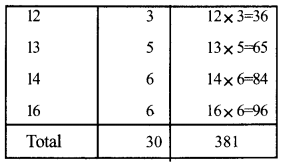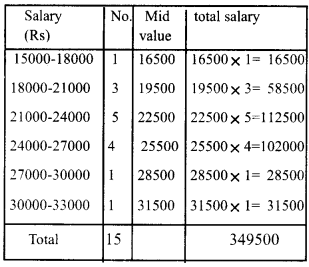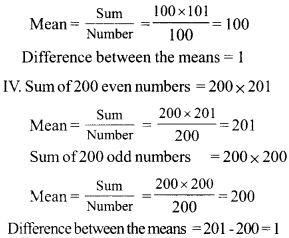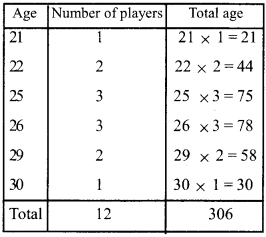# Kerala Syllabus 9th Standard Maths Solutions Chapter 13 Statistics

## Kerala State Syllabus 9th Standard Maths Solutions Chapter 13 Statistics

### Statistics Textual Questions and Answers

Textbook Page No. 193

Statistics Class 9 Kerala Syllabus Question 1.
The weight of 6 players in a volleyball team are all different and the average weight is 60 kilograms.
i. Prove that the team has at least one player weighing more than 60 kilograms.
ii. Prove that the team has at least one player weighing less than 60 kilograms.
i. Total weight of 6 players = 60 × 6 = 360 kg
The team contains players having weights 60, less than 60 or greater than 60. If the weights of all the players are less than 60, the average will also be less than 60. This is not possible. Therefore there will be at least one player having weight greater than 60.

ii. If the weight of all the players are greater than 60, the average will also be greater than 60. Therefore there will be at least one player having weight less than 60.

Hss Live Guru 9th Maths Kerala Syllabus Question 2.
Find two sets of 6 numbers with average 60, satisfying each of the conditions below:
i. 4 of the numbers are less than 60 and 2 of them greater than 60.
ii. 4 of the numbers are greater than 60 and 2 of them less than 60.
Total sum = 60 × 6 = 360
i. 20, 30, 40, 50, 100, 120

ii. 5, 15, 70, 80, 90, 100
Other ways are also possible.

Kerala Syllabus 9th Standard Maths Notes Question 3.
The table shows the children in a class, sorted according to the marks they got for a math test.

 Marks Children 2 1 3 2 4 5 5 4 6 6 7 11 8 10 9 4 10 2

Calculate the average marks of the class.
Total number of children is 45. Repeated addition can be written as multiplication.Average mark = $$\frac { Total }{ Number }$$ = $$\frac { 297 }{ 45 }$$ = 6.6

Class 9 Maths Solutions Kerala Syllabus Question 4.
The table below shows the days in a month sorted according to the amount of rainfall in a locality

 Rainfall(mm) Days 54 3 56 5 58 6 55 3 50 2 47 4 44 5 41 2

What is the average rainfall per day during this month?

 Rainfall(mm) Days Total 54 3 54 × 3 = 162 56 5 56 ×5 = 280 58 6 58 × 6 = 348 55 3 55 × 3 = 165 50 2 50 × 2=100 47 4 47 × 4 =188 44 5 44 × 5 = 220 41 2 41 × 2 = 82 Total 30 1545

The average of the rain fall per day during that month = $$\frac { Total rain fall }{ Number of days }$$
= $$\frac { 1545 }{ 30 }$$ = 51.5mm

Hsslive Guru 9th Maths Kerala Syllabus Question 5.
The details of rubber sheets a farmer got during a month are given below.

 Rubber (Kg) Days 9 3 10 4 11 3 12 3 13 5 14 6 16 6

i. How many kilograms of rubber did he get a day on average in this month?
ii. The price of rubber is 120 rupees per kilogram. What is his average income per day this month from selling rubber?i. Average Quantity of rubber per day = $$\frac { 381 }{ 30 }$$ = 12.77kg
ii. . If the price is Rs. 120 per kg, then average incomeperday = 12.7 × 120 = Rs.1524

Textbook Page No. 197

Kerala Syllabus 9th Standard Maths Solutions Question 1.
Find different sets of 6 different numbers between 10 and 30 with each number given below as mean:
i. 20
ii. 15
iii. 25
i. The mean of 6 numbers is 20.
ie; sum = 6 x 20= 120 (Write 3 pairs with sum 40)
i.e., 15, 25, 18, 22, 19, 21

ii. The mean of 6 numbers is 15
i.e; sum =6 x 15=90
(Write 30 pairs with sum 3)
12, 18, 13, 17, 14, 16

iii. Mean is 25
sum = 25 x 6 = 150
(Write 50 pairs with sum 3)
22, 28, 23, 27, 24, 26

Hss Live Guru Class 9 Maths Kerala Syllabus Question 2.
The table below shows the children in a class, sorted according to their heights.

 Height(cm) Number of children 148 – 152 8 152 – 156 10 156 – 160 15 160 – 164 10 164 – 168 7

What is the mean height of a child in this class?

 Height (cm) No. of children Mid Value Total Height 148 -152 8 150 150 × 8 = 1200 152 -156 10 154 154 × 10 = 1540 156 -160 15 158 158 × 15 = 2370 160 -164 10 162 162 × 10 = 1620 164 -168 7 166 160 × 7 = 1162 Total 50 7892

Mean height = $$\frac { Total height }{ No of children }$$
= $$\frac { 7892 }{ 50 }$$ = 157.84 cm

Hss Live Class 9 Maths Kerala Syllabus Question 3.
The teachers in a university are sorted according to their ages, as shown below.

 Age Number of Persons 25 – 30 6 30 – 35 14 35 – 40 16 40 – 45 22 45 – 50 5 50 – 55 4 55 – 60 3

What is the mean age of a teacher in this university?

 Age No.of persons Midvalue Total 25 – 30 6 27.5 165 30 – 35 14 32.5 455 35 – 40 16 37.5 600 40 – 45 22 ‘42.5 935 45 – 50 5 47.5 237.5 50 – 55 4 52.5 210 55 – 60 3 57.5 172.5 Total 70 2775

Mean age = $$\frac { Total age }{ No of persons }$$
= $$\frac { 2775 }{ 70 }$$ = 39.64

Kerala Syllabus 9th Standard Maths Question 4.
The table below shows children in a class sorted according to their weights.

 Weight (kg) Number of children 21 – 23 4 23 – 25 — 25 – 27 7 27 – 29 6 29 – 31 3 31 – 33 1

The mean weight is calculated as 26 kilograms. How many children have weights between 23 and 25 kilograms?
Let’s prepare the table for finding the mean by considering the number of children in the group 23 to 25, as ‘x’.

 Weight (kg) No.of children Mid value Total weight 21 – 23 4 22 22 × 4 = 88 23 – 25 X 24 24 × x = 24x 25 – 27 7 26 26 × 7 = 182 27 – 29 6 28 28 × 6 = 168 29 – 31 3 30 30 × 3 = 90 31 – 33 1 32 32 × 1 = 32 Total 21 + x 560 + 24x

Mean weight = 26 kgi.e; the number of children having weight between 23 to 25 is 7.

### Statistics Exam oriented Questions and Answers

Kerala Syllabus Std 9 Maths Solutions Question 1.
The details of rubber sheets got for a month by a farmer are given in the table.

 Rubber (kg) No. of days 7 3 8 4 9 5 10 6 11 ? 12 4 13 3

During this month he got an average of 10 sheets per day. If so in how many days did he get 11kg per day ?

 Rubber (kg) Days Total weights (kg) 7 3 7 × 3 = 21 8 4 8 × 4= 32 9 5 9 × 5 = 45 10 6 10 × 6 = 60 11 X 11 × x= 11x 12 4 12 × 4 = 48 13 3 13 × 3 = 39 Total 25 + x 245 + 11x

Let ‘x’ be the number of days in which he got 11 kg rubber sheet.∴ He got 11 kgs of sheets for 5 days.

Kerala Syllabus 9th Standard Maths Notes Malayalam Medium Question 2.
In a factory, there are workers belonging to four categories. The average income and the number of workers in each category are given. What is the mean income when all the workers in the four categories are combined?

 Class No.of workers Average income (Rs) I 12 6000 II 16 8000 III 8 9500 IV 4 11000Mean income = $$\frac { 320000 }{ 40 }$$
= Rs. 8000

Kerala Syllabus 9th Standard Maths Solution Question 3.
The daily wages of 10 workers in a factory are given below.
400, 350, 450, 500, 400, 500, 350, 500, 350, 450
If one more person is joined, the mean becomes Rs. 450. What is the daily wage of the new person?
Total wages of 10 workers = 4250 Total wages of 11 workers= 11 × 450 = Rs. 4950
Wage of the 11th person = 4950 – 4250

Kerala State Class 9 Maths Solutions Question 4.
Find 10 different numbers between 10 and 30 whose mean is 20.
Given mean is 20
Sum 20 × 10 = 200.
We have to find 10 different numbers whose sum is 200 (for this find 5 pairs of sum 40)
(15, 25) (16, 24) (17, 23) (18, 22) (19, 21)
The numbers are 15, 16, 17, 18, 19, 21, 22, 23, 24, 25

9th Maths Notes Kerala Syllabus Question 5.
A table categorizing the workers in an office on the basis of their salary is given below.

 Salary (Rs) Number of workers 15000 -18000 1 18000 – 21000 3 21000 – 24000 5 24000 – 27000 4 27000 – 30000 1 30000 – 33000 1

Find the mean of salary.Mean income = $$\frac { 349500 }{ 15 }$$
= R.s 23300

Kerala Syllabus 9th Standard Notes Maths Question 6.
i. Find the mean of natural numbers from 1 to 100.
ii. What is the mean of even numbers from 1 to 100? What is the mean of odd numbers?
iii. What is the difference between the means of the first 100 even numbers and odd numbers?
iv. What is the difference between the means of the first 200 even numbers and 200 odd numbers?
Sum of the natrural numbers from 1 to n = $$\frac n{ n + 1 }{ 2 }$$Sum of the first 100 odd numbers
= 1002 = 100 × 100In general, the difference between the means of n even numbers and n odd numbers is always 1.

Question 7.
A table tabulating the players in a cricket team on the basis of their age is given below.

 Age Number of players 21 1 22 2 25 3 26 3 29 2 30 1

Calculate the mean age of the players?